# Coordinates

Also found in: Dictionary, Thesaurus, Medical, Idioms, Wikipedia.

## coordinates

[kō′ȯrd·ən·əts]
(mapping)
Linear or angular quantities which designate the position that a point occupies in a given reference frame or system.
A general term to designate the particular kind of reference frame or system, such as plane rectangular coordinates or spherical coordinates.
(mathematics)
A set of numbers which locate a point in space.
McGraw-Hill Dictionary of Scientific & Technical Terms, 6E, Copyright © 2003 by The McGraw-Hill Companies, Inc.
The following article is from The Great Soviet Encyclopedia (1979). It might be outdated or ideologically biased.

## Coordinates

in geodesy, the set of three numbers that determines the position of a point on the earth’s surface relative to a particular initial surface. The initial, or reference, surface is the surface that in a particular approximation replaces the surface of the geoid. Depending on the purpose, the reference sur-face may be a plane (in topography this is the plane of the Gauss-Krueger projection), a sphere (the surface of the globe), or the surface of the reference ellipsoid.

The geodetic coordinates of a point are latitude B (the angle formed by the line normal to the ellipsoid passing through the given point and the plane of the ellipsoid’s equator), longitude L (the angle between the planes of the meridian of the given point and the prime meridian), and height H (the distance between the given point and the ellipsoid along the normal line). Geodetic coordinates cannot be obtained directly from observation, but they can be computed for any point included in a geodetic grid using data from geodetic measurements.

The astronomical coordinates of a point are latitude ϕ, or angle formed by a plumb line at the given point and the plane of the earth’s equator, and longitude λ, or angle between the planes of the astronomical meridians of the given point and the prime meridian. Thus, the above astronomical coordinates ø and λ are also called geographic coordinates. The normal height Hγ (the distance between the given point and the quasi-geoid along a plumb line), which is frequently equated with the elevation of the point above sea level, is added to ø and λ. The astronomical coordinates ø and λ are obtained from astronomical observations; the elevations of points on the earth’s surface are obtained by leveling. The geodetic coordinates of a particular point differ from the astronomical coordinates of the point owing to the selection of the ellipsoid and to the fact that the plumb line does not coincide with the line normal to the ellipsoid. A comparison of the geodetic and astronomical coordinates of a number of points on the earth’s surface makes it possible to study the surface of the geoid (more accurately the quasi-geoid) in a given sector relative to the ellipsoid employed (astronomical leveling and astronomical-gravimetric leveling).

Other types of coordinates are also used in geodesy. With the development of space geodesy the rectangular geodetic coordinates X, y, and Z, whose origin O coincides with the center of the ellipsoid and whose axis Z is directed along the ellipsoid’s lesser axis, have become very important. The transition from B, L, and H to X, Y, and Z is made by means of fairly simple formulas.

Various curvilinear coordinates on the surface of ellipsoids are also used in studying many questions of geodesy. In practice, when using geodetic data and topographical maps, rectangular coordinates on the plane of a geodetic projection are employed.

### REFERENCES

Krasovskii, F. N. Rukovodstvo po vysshei geodezii, part 2. Moscow, 1942.
Zakatov, P. S. Kurs vysshei geodezii, 3rd ed. Moscow, 1964.
Morozov, V. P. Kurs sferoidicheskoi geodezii. Moscow, 1969.
Grushinskii, N. P. Teoriia figury Zemli. Moscow, 1963.

G. A. MESHCHERIAKOV[13--347–1]

## Coordinates

numbers that determine the position of a point in the plane, on a surface, or in space. Astronomical and geographical (spherical) coordinates—latitude and longitude— which locate a point on the celestial sphere or on the surface of the earth, were the first types of coordinates to be systematically used. In the 14th century the French mathematician N. Oresme used coordinates in the plane to construct graphs, using the terms longitude and latitude for what we now call abscissa and ordinate.

Coordinates were more systematically used in problems of plane geometry in the 17th century. The French scientist R. Descartes is credited with elucidating the full significance of the method of coordinates, which has made it possible to trans-late geometric problems systematically into the language of mathematical analysis and, conversely, to interpret the facts of analysis geometrically.

In addition to coordinates of a point, coordinates of a line, plane, and other geometric objects are also considered. Coordinates of mechanical systems, which are numbers that determine the position of a mechanical system (for example, a rigid body) at each moment of time, are used in theoretical mechanics.

Coordinates of a point in the plane. Affine, or general Carte-sian, coordinates of a point in the plane are obtained by selecting a point O (origin) and two vectors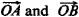not lying on the same line and issuing from O. The position of a point P is determined (in the chosen coordinate system) by two coordinates, the abscissa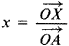and the ordinate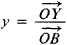where XP is parallel to OB and YP is parallel to OA (see Figure 1, where x = 2, y = — 1).

In the particular case when the vectorsare perpendicular and have the same length, we speak of rectangular coordinates. Such coordinates are the most frequently used. If the angle betweenis arbitrary but the lengths of the two vectors are equal, than we speak of oblique coordinates, to which Descartes himself limited his study (often only oblique coordinates are called Cartesian coordinates, the term “affine coordinates” being reserved for general Cartesian coordinates).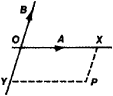Figure 1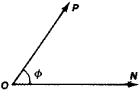Figure 2

The polar coordinates of a point in the plane are obtained by selecting a point O (the pole), a ray ON issuing from it (see Figure 2), and a unit of measure for length. The distance p = OP and the angle ø = ≮ NOP serve as the coordinates of the point P. In order to associate to every point P in the plane a pair of numbers (p, ø), it is sufficient to consider values of p and ø subject to the inequalities 0 ≤ p < ∞ and 0 ≤ ø < . With the exception of the point O, for which p = 0 and the angle ø is not defined, the correspondence between the points P different from O and the pairs (p, ø), subject to the indicated conditions, is one-to-one.

Among other specialized coordinate systems in the plane, elliptic coordinates should be noted.

In the case of affine coordinates, the lines x = const form a pencil of lines that are parallel to the Oy axis, while the lines y — const form another pencil of lines that are parallel to the Ox axis. One line of the first pencil (x — x0) and one line from the second pencil (y — y0) pass through every point P (x0, y0) in the plane. In the case of polar coordinates, the curves p = const are circles and the curves ø = const are rays issuing from the origin O. Exactly one curve of each family passes through every point P different from O. The numbers po and ø associated with these two curves are the coordinates of the point P. More generally, we may consider two functions u(P) and υ(P) of a point in a region G of the plane, such that every curve u(P) = const intersects every curve υ(P) = const in just one point of G. Then the numbers u(P) and υ(P) uniquely determine the position of the point P in (7, that is, they are the coordinates of P. The curves determined by the equations u = const and υ = const are called coordinate curves.

Curvilinear coordinates on a surface. The above concept can be used without change to introduce curvilinear coordinates on an arbitrary surface. For example, on a sphere where (ø is longitude and 0 is latitude, the curves ø = const are the meridians and the curves 0 = const are the latitude circles, whose distribution is familiar from the elements of geography. Curvilinear, or Gaussian, coordinates on an arbitrary surface are the fundamental apparatus of the differential geometry of surfaces.

Homogeneous coordinates in the plane. A Euclidean plane supplemented with infinitely distant elements can be considered from the projective standpoint as a closed surface on which the infinitely distant points do not play any special role. It is impossible to introduce coordinates in the entire projective plane that would characterize the position of a point by a number pair (u, υ) with preservation of the one-to-one nature and continuity of the correspondence. Homogeneous coordinates are used in-stead. In this case, each point is made to correspond not to a pair but to a triple of numbers (x1, X2, x3); moreover, the same point corresponds to two triples (x1 x2, x3) and (x1’, x2’ x3) if and only if the triples are proportional, that is, there exists a factor A such that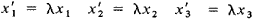These coordinate systems play an important role in geometry.

Coordinates of a point in space. Affine, or general Cartesian, coordinates in three-dimensional space are introduced by specifying a point O and three vectors ex =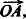, ey =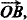, and ez =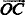not all lying in the same plane. In order to obtain the x, y, z coordinates of a point P, the vector OP is represented in the form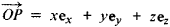In the simplest case of rectangular coordinates, the vectors ex, ey, and ez are pairwise perpendicular and are each of unit length. Two essentially different types of rectangular coordinate systems in space are possible, namely a right-handed system (see Figure 3, where ey and er lie in the plane of the drawing and ex is directed toward the reader) and a left-handed system (see Figure 4, where ex and ez lie in the plane of the drawing and ey is directed toward the reader).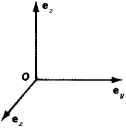Figure 3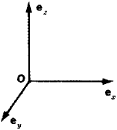Figure 4

Curvilinear coordinate systems are also used in space. Specifically, in some region G of space, three functions of a point u(P), ν(P), and w(P) are considered that obey the condition that one surface of the family u — const, one surface of the family ν = const, and one surface of the family w = const pass through a point P of G. Each point is thereby associated to three numbers (u, ν, w), which are its coordinates. The surfaces defined by the equations u = const, ν = const, or w = const are all called coordinate surfaces.

In applications to mechanics, mathematical physics, and other fields, certain special curvilinear coordinate systems, namely, spherical coordinates and cylindrical coordinates, are the most widely used.

Coordinates of a line, plane, and so forth. The principle of duality (seeDUALITY, PRINCIPLE OF), which establishes the equivalence of points and lines in the geometry of two dimensions and the equivalence of points and planes in the geometry of three dimensions, suggests the notion of determining the positions of lines and planes by means of special coordinates. In fact, if the equation of a line (not passing through the origin) in rectangular coordinates is reduced to the form ux + νy + 1 =0, then the position of the line is completely determined by the numbers u and ν (u = — 1/a and ν = —1/b, where a and b are the intercepts of the line on the axes). We may take (u, ν) to be the coordinates (known as the tangential coordinates) of the straight line. The symmetrical nature of the equation ux + νy + 1= 0 with respect to the pairs (x, y) and (u, ν) is an analytic expression of the principle of duality. The use of coordinates in n-dimensional space is entirely analogous to the cases n = 2 (plane or surface) and n = 3 (three-dimensional space).

A. N. KOLMOGOROV

## coordinates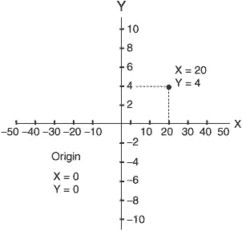Two-dimensional system of coordinates.
A system in which two magnitudes are used to define a position. Usually expressed in degrees/minutes/seconds of latitude and longitude. These may be interrelated linear or angular measures, by which the position of a point may be defined with reference to a fixed axis, a place, or a direction. Coordinates may also be three-dimensional as in the Cartesian system.
References in periodicals archive ?
Fallacy 3 is an attempt to find coordinates that will show the particle can reach and cross the critical radius.
It is Equation 3 that indicates the certain function relation between the bed coordinates and the diagram layer coordinates.
Coordinates in the default camera coordinate system (units: pixels) are shown in Table 1.
Based on the coordinates of six spatial feature points and the orthogonal constraint condition of the rotation matrices, the rotation matrix and the translation matrix of the two-theodolite system are obtained as follows:
The Cooper's iterative method is used to calculate the optimal coordinates for distribution warehouse allocation so that the city which is the closest to that coordinates becomes a good place for allocating a distribution warehouse.
The post processed coordinates of YLDZ station were entered into the Ashtech web server software to be used in real-time measurements in order to determine the coordinates of benchmark points in the next stage.
The First Vice Prime Minister will coordinate interethnic relations, reforms of administrative and territorial system, activities of local state administrations, local government bodies.
Since the initial virtual supervisors mobile robot coordinates are known, during the procedure of supervisor's coordinate conversion, the chosen optimization method, it is easy to find and the actual coordinates of the robot, but it is unclear how much time will have to spend for this solution.
In some mines of the OKR, Ott's system of coordinates was still in use in 1970s; even today, its practical value consists in the ability to trace and dispose of old mine outlets or test and verify pits surveyed with the use of this system.
Improved APIT algorithm , which bases on triangle-gravity-center calculation and grid scanning, considers the triangle-gravity-center as the coordinates of unknown nodes.

Site: Follow: Share:
Open / Close